# JP3628334B2 - Tuning amplifier - Google Patents

## Info

Publication number
JP3628334B2
JP3628334B2 JP51804497A JP51804497A JP3628334B2 JP 3628334 B2 JP3628334 B2 JP 3628334B2 JP 51804497 A JP51804497 A JP 51804497A JP 51804497 A JP51804497 A JP 51804497A JP 3628334 B2 JP3628334 B2 JP 3628334B2
Authority
JP
Japan
Prior art keywords
phase shift
resistor
circuit
input
tuning
Prior art date
Legal status (The legal status is an assumption and is not a legal conclusion. Google has not performed a legal analysis and makes no representation as to the accuracy of the status listed.)
Expired - Fee Related
Application number
JP51804497A
Other languages
Japanese (ja)
Inventor

Original Assignee

Priority date (The priority date is an assumption and is not a legal conclusion. Google has not performed a legal analysis and makes no representation as to the accuracy of the date listed.)
Filing date
Publication date
Priority to JP31367095 priority Critical
Priority to JP31366995 priority
Application filed by 池田 毅 filed Critical 池田 毅
Priority to PCT/JP1996/000530 priority patent/WO1997017754A1/en
Application granted granted Critical
Publication of JP3628334B2 publication Critical patent/JP3628334B2/en
Anticipated expiration legal-status Critical
Application status is Expired - Fee Related legal-status Critical

## Images

•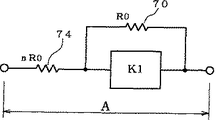•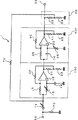•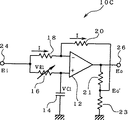•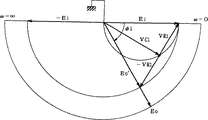•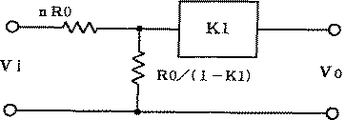•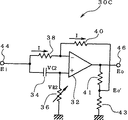•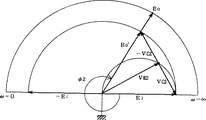••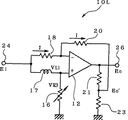••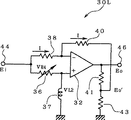•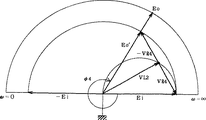•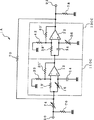•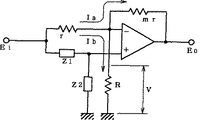•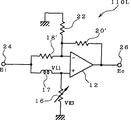•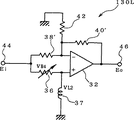•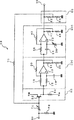•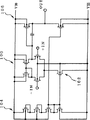## Classifications

• HELECTRICITY
• H03BASIC ELECTRONIC CIRCUITRY
• H03HIMPEDANCE NETWORKS, e.g. RESONANT CIRCUITS; RESONATORS
• H03H11/00Networks using active elements
• H03H11/02Multiple-port networks
• H03H11/16Networks for phase shifting
• H03H11/20Two-port phase shifters providing an adjustable phase shift
• HELECTRICITY
• H03BASIC ELECTRONIC CIRCUITRY
• H03FAMPLIFIERS
• H03F3/00Amplifiers with only discharge tubes or only semiconductor devices as amplifying elements
• H03F3/189High frequency amplifiers, e.g. radio frequency amplifiers
• H03F3/19High frequency amplifiers, e.g. radio frequency amplifiers with semiconductor devices only
• H03F3/191Tuned amplifiers
• HELECTRICITY
• H03BASIC ELECTRONIC CIRCUITRY
• H03FAMPLIFIERS
• H03F3/00Amplifiers with only discharge tubes or only semiconductor devices as amplifying elements
• H03F3/189High frequency amplifiers, e.g. radio frequency amplifiers
• H03F3/19High frequency amplifiers, e.g. radio frequency amplifiers with semiconductor devices only
• H03F3/195High frequency amplifiers, e.g. radio frequency amplifiers with semiconductor devices only in integrated circuits
• HELECTRICITY
• H03BASIC ELECTRONIC CIRCUITRY
• H03HIMPEDANCE NETWORKS, e.g. RESONANT CIRCUITS; RESONATORS
• H03H11/00Networks using active elements
• H03H11/02Multiple-port networks
• H03H11/04Frequency selective two-port networks
• H03H11/12Frequency selective two-port networks using amplifiers with feedback
• H03H11/1217Frequency selective two-port networks using amplifiers with feedback using a plurality of operational amplifiers

## Description

Technical field
The present invention relates to a tuning amplifier that can be easily integrated, and more particularly to a tuning amplifier that can arbitrarily adjust a tuning frequency and a maximum attenuation amount without interfering with each other.
Background art
Conventionally, various tuning amplifiers using active elements and reactance elements have been proposed and put into practical use.
For example, in a conventional tuning amplifier using LC resonance, Q and gain depending on the LC circuit change when the tuning frequency is adjusted, the tuning frequency changes when the maximum attenuation is adjusted, and at the tuning frequency when the maximum attenuation is adjusted. It has the feature that the gain changes. Therefore, in the conventional tuning amplifier, it is extremely difficult to adjust the tuning frequency, the gain at the tuning frequency, and the maximum attenuation amounts C1 and C2 without interfering with each other. In addition, it has been difficult to form a tuning amplifier that can adjust the tuning frequency and the maximum attenuation amount by an integrated circuit.
The present invention was created in view of the above points, and its purpose is suitable for integration, and it is possible to adjust the tuning frequency, the gain at the tuning frequency, and the maximum attenuation without interfering with each other. In particular, it is an object of the present invention to provide a tuning amplifier that can suppress a change in output amplitude when the tuning frequency is varied.
Disclosure of the invention
The present invention has been conceived to solve such a problem, and the tuning frequency, the gain at the tuning frequency, and the maximum attenuation can be adjusted without interfering with each other, particularly when the tuning frequency is varied. An object of the present invention is to provide a tuning amplifier capable of suppressing a change in output amplitude.
The tuning amplifier of the present invention includes two all-pass type phase shift circuits including a differential amplifier whose output is fed back to the input side,
A voltage dividing circuit inserted into a part of a feedback loop formed by cascading each of the two phase shift circuits,
The signal added by the adder circuit is input to the previous phase shift circuit of the two cascaded phase shift circuits, and the signal output from the subsequent phase shift circuit is used as the feedback signal as the feedback signal. An AC signal input to the circuit and input to the voltage dividing circuit is output as the tuning signal.
The tuned amplifier of the present invention includes an input impedance element in which an input signal is input to one end and a feedback impedance element in which a feedback signal is input to one end, and the input signal and the feedback signal are An adding circuit for adding;
A differential amplifier in which one end of a first resistor is connected to an inverting input terminal and an AC signal is input through the first resistor, and a first voltage dividing circuit connected to the output terminal of the differential amplifier And a second resistor connected between the output terminal of the first voltage dividing circuit and the inverting input terminal of the differential amplifier, a reactance element such as a capacitor or an inductor, and a third resistor. Two phase shift circuits including a series circuit connected to the other end of the first resistor, and connecting a connection portion of the third resistor and the reactance element to a non-inverting input terminal of the differential amplifier;
Each of the two phase shift circuits is connected in cascade, and the signal added by the adder circuit is input to the previous phase shift circuit among the two phase shift circuits connected in cascade. The signal output from the phase shift circuit is input to the one end of the feedback impedance element as the feedback signal, and the output of either of these two phase shift circuits is output as the tuning signal.
The tuned amplifier of the present invention includes an input impedance element in which an input signal is input to one end and a feedback impedance element in which a feedback signal is input to one end, and the input signal and the feedback signal are An adding circuit for adding;
A differential amplifier to which one end of the first resistor of the inverting input terminal is connected and an AC signal is input via the first resistor, and is connected between the inverting input terminal and the output terminal of the differential amplifier. A second resistor, a third resistor having one end connected to the inverting input terminal of the differential amplifier and the other end grounded, a reactance element such as a capacitor or an inductor, and a fourth resistor. Two phase shift circuits including a series circuit connected to the other end of the first resistor, and a connection portion of the fourth resistor and the reactance element connected to a non-inverting input terminal of the differential amplifier;
Each of the two phase shift circuits is connected in cascade, and the signal added by the adder circuit is input to the previous phase shift circuit among the two phase shift circuits connected in cascade. The signal output from the phase shift circuit is input to the one end of the feedback impedance element as the feedback signal, and the output of either of these two phase shift circuits is output as the tuning signal.
The tuned amplifier of the present invention includes an input impedance element in which an input signal is input to one end and a feedback impedance element in which a feedback signal is input to one end, and the input signal and the feedback signal are An adding circuit for adding;
A differential amplifier in which one end of a first resistor is connected to an inverting input terminal and an AC signal is input through the first resistor, and a first voltage dividing circuit connected to the output terminal of the differential amplifier And a second resistor connected between the output terminal of the first voltage dividing circuit and the inverting input terminal of the differential amplifier, a reactance element such as a capacitor or an inductor, and a third resistor. A first phase shift circuit including a series circuit connected to the other end of the first resistor, and a connection portion of the third resistor and the reactance element connected to a non-inverting input terminal of the differential amplifier;
A differential amplifier in which one end of a fourth resistor is connected to the inverting input terminal and an AC signal is input through the fourth resistor, and is connected between the inverting input terminal and the output terminal of the differential amplifier. A fifth resistor, a sixth resistor having one end connected to the inverting input terminal of the differential amplifier and the other end grounded, a reactance element such as a capacitor or an inductor, and a seventh resistor. A series circuit connected to the other end of the four resistors, and a second phase shift circuit in which a connection portion of the seventh resistor and the reactance element is connected to a non-inverting input terminal of the differential amplifier;
The first and second phase shift circuits are connected in cascade, and the signal added by the adder circuit is input to the previous phase shift circuit of the two phase shift circuits connected in cascade. The signal output from the subsequent phase shift circuit is input to the one end of the feedback impedance element as the feedback signal, and the output of one of the first and second phase shift circuits is output as the tuning signal.
[Brief description of the drawings]
FIG. 1 is a circuit diagram showing a configuration of a tuning amplifier according to a first embodiment to which the present invention is applied;
FIG. 2 is a diagram showing an extracted configuration of the previous phase shift circuit shown in FIG.
FIG. 3 is a vector diagram showing the relationship between the input / output voltage of the previous phase shift circuit and the voltage appearing on the capacitor,
FIG. 4 is a diagram showing the configuration of the subsequent phase shift circuit shown in FIG.
FIG. 5 is a vector diagram showing the relationship between the input / output voltage of the subsequent phase shift circuit and the voltage appearing on the capacitor,
FIG. 6 is a system diagram in which two phase shift circuits and voltage divider circuits having the above-described configuration are replaced with a circuit having a transfer function K1,
FIG. 7 is a system diagram in which the system shown in FIG. 6 is replaced by the mirror theorem.
FIG. 8 is a diagram showing the tuning characteristics of the tuning amplifier shown in FIG.
FIG. 9 is a circuit diagram showing a configuration of a phase shift circuit including an LR circuit,
FIG. 10 is a vector diagram showing the relationship between the input / output voltage of the phase shift circuit shown in FIG. 9 and the voltage appearing in the inductor, etc.
FIG. 11 is a circuit diagram showing the configuration of another phase shift circuit including the LR circuit,
FIG. 12 is a vector diagram showing the relationship between the input / output voltage of the phase shift circuit shown in FIG. 11 and the voltage appearing in the inductor,
FIG. 13 is a circuit diagram showing a third embodiment of the tuning amplifier;
FIG. 14 is a generalized diagram of the phase shift circuit shown in FIG.
FIG. 15 is a circuit diagram showing the configuration of a phase shift circuit that can be replaced with the phase shift circuit in the previous stage of the tuning amplifier shown in FIG.
FIG. 16 is a circuit diagram showing a configuration of a phase shift circuit that can be replaced with a phase shift circuit in the subsequent stage of the tuning amplifier shown in FIG.
FIG. 17 is a circuit diagram showing a configuration of a fifth embodiment of a tuning amplifier;
FIG. 18 is a circuit diagram in which a portion necessary for the operation of the phase shift circuit of the present invention is pushed out in the configuration of the operational amplifier.
BEST MODE FOR CARRYING OUT THE INVENTION
[First Embodiment of Tuning Amplifier]
FIG. 1 is a circuit diagram showing a configuration of a tuning amplifier according to a first embodiment to which the present invention is applied.
The tuning amplifier 1 shown in the figure includes two phase shift circuits 10C and 30C that perform a total phase shift of 360 ° at a predetermined frequency by shifting the phase of an AC signal to be input by a predetermined amount, A voltage dividing circuit 60 comprising resistors 62 and 64 provided on the output side of the phase shift circuit 30C, a feedback resistor 70 and an input resistor 74 (the input resistor 74 has a resistance value n times the resistance value of the feedback resistor 70) And an adder circuit that adds the divided output (feedback signal) of the voltage dividing circuit 60 and the signal (input signal) input to the input terminal 90 at a predetermined ratio. It consists of
FIG. 2 shows an extracted configuration of the preceding phase shift circuit 10C shown in FIG. The phase shift circuit 10C shown in the figure includes a variable resistor 16 and a capacitor 14 that shift the phase of the AC signal input to the input terminal 24 by a predetermined amount and input to the non-inverting input terminal of the operational amplifier 12, and the input terminal 24 and the operational amplifier. A resistor 18 inserted between the inverting input terminal of 12, resistors 21 and 23 connected to the output terminal of the operational amplifier 12 to form a voltage dividing circuit, and the inverting input terminal of the voltage dividing circuit and the operational amplifier 12. And a resistor 20 connected therebetween.
In the phase shift circuit 10C having such a configuration, the resistance values of the resistors 18 and 20 are set to be the same.
When a predetermined AC signal is input to the input terminal 24 shown in FIG. 2, the voltage VC1 appearing across the capacitor 14 is applied to the non-inverting input terminal of the operational amplifier 12. Further, at the both ends of the resistor 18, the same voltage VR1 as the voltage VR1 appearing at both ends of the variable resistor 16 appears. The same current I flows through the two resistors 18 and 20, and since the resistance values of the resistors 18 and 20 are equal as described above, the voltage VR1 also appears across the resistor 20. Considering the inverting input terminal (voltage VC1) of the operational amplifier 12 as a reference, the voltage obtained by adding the voltage VR1 across the resistor 18 as a vector is the vector voltage obtained by subtracting the voltage VR1 across the resistor 20 from the input voltage Ei. The voltage at the connection point between the resistor 21 and the resistor 23 (divided voltage output) Eo ′.
FIG. 3 is a vector diagram showing the relationship between the input / output voltage of the preceding phase shift circuit 10C and the voltage appearing in the capacitor or the like.
As described above, when the voltage VC1 applied to the non-inverting input terminal of the operational amplifier 12 is considered as a reference, the input voltage Ei and the divided voltage Eo ′ differ only in the direction in which the voltage VR1 is synthesized, and the absolute value thereof is Will be equal. Therefore, the relationship between the magnitude and phase of the input voltage Ei and the divided output Eo ′ can be expressed by an isosceles triangle with the input voltage Ei and the divided output Eo ′ as the hypotenuse and the base of twice the voltage VR1. It can be seen that the amplitude of the divided voltage output Eo ′ is the same as the amplitude of the input signal regardless of the frequency, and the phase shift amount is represented by φ1 shown in FIG. The phase shift amount φ1 varies from 0 ° to 180 ° depending on the frequency. In addition, the phase shift amount φ1 is changed by changing the resistance value R of the variable resistor 16.
Since the output terminal 26 of the phase shift circuit 10C is connected to the output terminal of the operational amplifier 12, assuming that the resistance value of the resistor 21 is R21 and the resistance value of the resistor 23 is R23, the output voltage Eo and the above-described divided output Eo ′ has a relationship of Eo = (1 + R21 / R23) Eo ′ when R21 and R23 are sufficiently small with respect to the resistance value of the resistor 20. Therefore, a gain larger than 1 can be obtained by adjusting the values of R21 and R23, and the amplitude of the output voltage Eo is constant even when the frequency is changed as shown in FIG. 3, and only the phase is shifted by a predetermined amount. can do.
Similarly, FIG. 4 shows an extracted configuration of the subsequent phase shift circuit 30C shown in FIG. The latter phase shift circuit 30C shown in the figure includes an operational amplifier 32, which is a kind of differential amplifier, and a capacitor that shifts the phase of the signal input to the input terminal 44 by a predetermined amount and inputs it to the non-inverting input terminal of the operational amplifier 32 34, the variable resistor 36, the resistor 38 inserted between the input terminal 44 and the inverting input terminal of the operational amplifier 32, the resistors 41 and 43 connected to the output terminal of the operational amplifier 32 to form a voltage dividing circuit, A resistor 40 connected between the voltage dividing circuit and the inverting input terminal of the operational amplifier 32 is included.
In the phase shift circuit 30C having such a configuration, the resistance values of the resistor 38 and the resistor 40 are set to be the same.
When a predetermined AC signal is input to the input terminal 44 shown in FIG. 4, the voltage VR2 appearing across the variable resistor 36 is applied to the non-inverting input terminal of the operational amplifier 32. Further, at the both ends of the resistor 38, the same voltage VC2 as the voltage VC2 appearing at both ends of the capacitor 34 appears. Since the same current I flows through the two resistors 38 and 40 and the resistance values of the resistors 38 and 40 are equal as described above, the voltage VC2 appears at both ends of the resistor 40. Considering the inverting input terminal (voltage VR2) of the operational amplifier 32 as a reference, the vector voltage of the both-ends voltage VC2 of the resistor 38 is added to the input voltage Ei and the voltage VC2 across the resistor 40 is subtracted in a vector form. The voltage at the connection point between the resistor 41 and the resistor 43 (divided voltage output) Eo ′.
FIG. 5 is a vector diagram showing the relationship between the input / output voltage of the subsequent phase shift circuit 30C and the voltage appearing in the capacitor or the like.
As described above, considering the voltage VR2 applied to the non-inverting input terminal of the operational amplifier 32 as a reference, the input voltage Ei and the divided output Eo ′ differ only in the direction in which the voltage VC2 is synthesized, and the absolute value thereof is Will be equal. Therefore, the relationship between the magnitude and phase of the input voltage Ei and the divided output Eo ′ can be represented by an isosceles triangle having the input voltage Ei and the divided output Eo ′ as the hypotenuse and the base of twice the voltage VC2. It can be seen that the amplitude of the divided voltage output Eo ′ is the same as the amplitude of the input signal regardless of the frequency, and the phase shift amount is represented by φ2 shown in FIG. This phase shift amount φ2 changes from 180 ° to 360 ° in the clockwise direction (phase delay direction) with reference to the input voltage Ei according to the frequency. In addition, by changing the resistance value R of the variable resistor 36, the phase shift amount φ2 changes.
Since the output terminal 46 of the phase shift circuit 30C is connected to the output terminal of the operational amplifier 32, if the resistance value of the resistor 41 is R41 and the resistance value of the resistor 43 is R43, the output voltage Eo and the above-described divided output Eo ′ has a relationship of Eo = (1 + R41 / R43) Eo ′ when R41 and R43 are sufficiently small with respect to the resistance value of the resistor 40. Therefore, a gain greater than 1 can be obtained by adjusting the values of R41 and R43, and the amplitude of the output voltage Eo is constant even when the frequency changes as shown in FIG. 5, and only the phase is shifted by a predetermined amount. can do.
In this way, the phase is shifted by a predetermined amount in each of the two phase shift circuits 10C and 30C, and as shown in FIGS. 3 and 5, the two phase shift circuits 10C and 30C at the predetermined frequency as a whole. The total amount of phase shift is 360 °.
Further, the output of the subsequent phase shift circuit 30C is taken out from the output terminal 92 as the output of the tuning amplifier 1, and a signal obtained by passing the output of the phase shift circuit 30C through the voltage dividing circuit 60 is passed through the feedback resistor 70 to the previous stage. Is fed back to the input side of the phase shift circuit 10C. Then, the fed back signal and the signal input via the input resistor 74 are added, and the added signal is input to the previous phase shift circuit 10C.
As described above, the total phase shift amount at a predetermined frequency is 360 ° by the two phase shift circuits 10C and 30C. At this time, the phase shift circuits 10C and 30C, the voltage dividing circuit 60, and the feedback resistor 70 are formed. By setting the loop gain of the feedback loop to be 1 or less, the tuning operation for passing only the signal of the predetermined frequency component described above is performed.
Further, since the output of the phase shift circuit 30C before being inputted to the voltage dividing circuit 60 is taken out from the output terminal 92 of the tuning amplifier 1, the tuning amplifier 1 itself can be given a gain, and the tuning operation can be performed. At the same time, the signal amplitude can be increased.
FIG. 6 is a system diagram in which the two phase shift circuits 10C and 30C having the above-described configuration and the voltage dividing circuit 60 are replaced with a circuit having a transfer function K1, and a resistor in parallel with the circuit having the transfer function K1. A feedback resistor 70 having R0 is connected in series with an input resistor 74 having a resistance value (nR0) n times that of the feedback resistor 70.
FIG. 7 is a system diagram in which the system shown in FIG. 6 is converted by Miller's theorem, and the transfer function A of the entire system after conversion is
A = Vo / Vi = K1 / {n (1-K1) +1} (1)
Can be expressed as
The transfer function K2 of the preceding phase shift circuit 10C is the time constant of the CR circuit composed of the variable resistor 16 and the capacitor 14 as T1(If the resistance value of the variable resistor 16 is R and the capacitance of the capacitor 14 is C, T1= CR)
K2 ＝ a1(1-T1s) / (1 + T1s) (2)
It becomes. Where s = jω and a1Is the gain of the phase shift circuit 10C, where a1= (1 + R21 / R23)> 1.
Further, the transfer function K3 of the subsequent phase shift circuit 30C is the time constant T of the CR circuit composed of the capacitor 34 and the variable resistor 36.2(If the capacitance of the capacitor 34 is C and the resistance of the variable resistor 36 is R, T2= CR)
K3 = -a2(1-T2s) / (1 + T2s) (3)
It becomes. Where a2Is the gain of the phase shift circuit 30C, and a2= (1 + R41 / R43)> 1.
The signal amplitude is 1 / a through the voltage divider circuit 60.1a2When the two phase shift circuits 10C, 30C and the voltage dividing circuit 60 are connected in cascade, the overall transfer function K1 is
K1 =-{1+ (Ts)2-2Ts} / {1+ (Ts)2+ 2Ts} (4)
It becomes. In the above equation (4), in order to simplify the calculation, the time constant T of each phase shift circuit is set.1, T2Both are T. Substituting this equation (4) into the above equation (1),
A =-{1+ (Ts)2−2Ts}
/ [(2n + 1) {1+ (Ts)2} + 2Ts]
=-{1 / (2n + 1)} [{1+ (Ts)2−2Ts}
/ {1+ (Ts)2+ 2Ts / (2n + 1)}] (5)
It becomes.
According to the equation (5), it can be seen that when ω = 0 (DC region), A = −1 / (2n + 1), and the maximum attenuation is given. It can also be seen that even when ω = ∞, A = −1 / (2n + 1), giving the maximum attenuation. Furthermore, the tuning point of ω = 1 / T (if the time constant of each phase shift circuit is different,
It can be seen that A = 1 at the tuning point) and is independent of the resistance ratio n of the feedback resistor 70 and the input resistor 74. In other words, as shown in FIG. 8, even if the value of n is changed, the tuning point does not shift and the attenuation at the tuning point does not change.
Incidentally, in FIG. 6 described above, when the all-pass circuit indicated by the transfer function K1 has an input impedance, a voltage dividing circuit is formed by the feedback resistor 70 and the input impedance of the all-pass circuit. The open loop gain of the feedback loop including it is smaller than the absolute value of the transfer function K1. The input impedance of the all-pass circuit is the input impedance of the preceding phase shift circuit 10C, and is formed by connecting the series resistance of the CR circuit composed of the variable resistor 16 and the capacitor 14 to the input resistor 18 of the operational amplifier 12 in parallel. It is none other than input impedance. Therefore, in order to compensate for the loss of the open loop gain of the feedback loop due to the input impedance of the all-pass circuit, it is necessary to set the gain of the all-pass circuit itself to 1 or more.
For example, the voltage dividing circuit by the resistors 21 and 23 included in the phase shift circuit 10C is ignored (the case where the voltage dividing ratio is 1 and a in the above-described equation (2)1In the range from the follower circuit having a gain of 1 to the inverting amplifier having a gain of -1 according to the input frequency, the phase shift circuit 10C Since it must operate, it is not preferable that the resistance ratio of the resistors 18 and 20 be other than 1. This is because when the resistance values of the resistors 18 and 20 are R18 and R20, the gain when the phase shift circuit 10C operates as an inverting amplifier is −R20 / R18, but the gain when the phase shift circuit 10C operates as a follower circuit is 18 Therefore, if the resistance ratio between the resistor 18 and the resistor 20 is not 1, only the phase between the input and output changes in the entire region where the phase shift circuit 10C operates. This is because the ideal condition in which the output amplitude does not change cannot be satisfied.
By adding a voltage dividing circuit consisting of a resistor 21 and a resistor 23 to the output side of the phase shift circuit 10C, and applying feedback to the inverting input terminal of the operational amplifier 12 through this voltage dividing circuit, the resistors 18 and 20 It is possible to set the gain of the phase shift circuit 10C to 1 or more while maintaining the ratio at 1. Similarly, a voltage dividing circuit composed of a resistor 41 and a resistor 43 is added to the output side of the phase shift circuit 30C, and feedback to the inverting input terminal of the operational amplifier 32 is performed via this voltage dividing circuit, so that the resistor 38 and the resistor It is possible to set the gain of the phase shift circuit 30C to 1 or more while maintaining the resistance ratio of 40 at 1.
As described above, by changing the resistance values of the variable resistor 16 in the phase shift circuit 10C and the variable resistor 36 in the phase shift circuit 30C, the time constant T of the CR circuit including the capacitor 14 and the variable resistor 16 is changed.1And the time constant T of the CR circuit composed of the capacitor 34 and the variable resistor 36.2Can be changed,
The tuning frequency ω calculated by can be varied within a certain range.
Further, when φ1 and φ2 shown in FIG. 3 and FIG. 5 are obtained from the equation (2) or (3),
φ1 = tan {2ωT1/ (1-ω2T1 2)} (6)
φ2 = tan {2ωT2/ (1-ω2T2 2)} (7)
It becomes. In the equations (6) and (7), φ1 and φ2 are obtained by setting the clockwise direction (phase delay direction) as the positive direction with reference to the input voltage Ei shown in FIGS.
For example T1= T2In the case of (= T), when ω = 1 / T, the total phase shift amount by the two phase shift circuits 10C and 30C is 360 °, and the above-described tuning operation is performed. At this time, φ1 = 90 °, φ2 = 270 °.
As described above, the tuning amplifier 1 of the first embodiment has a constant tuning frequency and a constant gain even when the resistance ratio n between the feedback resistor 70 and the input resistor 74 is changed, and has a maximum attenuation and tuning bandwidth. Since the tuning operation is performed using the tunable amplifier 1 that can be changed, when interference occurs, the above-described resistance ratio n can be set large to reduce the tuning bandwidth, thereby preventing interference. When there is little interference, the received signal can be faithfully reproduced by setting the above-described resistance ratio n small to widen the tuning bandwidth.
In the first embodiment described above, the resistance values of the feedback resistor 70 and the input resistor 74 are fixed, but at least one of the resistors can be varied to arbitrarily change the resistance ratio n described above. It may be possible.
In the tuning amplifier 1 of the first embodiment, the resistance values of the resistor 18 and the resistor 20 in the phase shift circuit 10C are set to the same value, and the resistance values of the resistor 38 and the resistor 40 in the phase shift circuit 30C are the same. Since the value is set to a value, the amplitude does not vary even if the tuning frequency is changed, and a tuning output having a substantially constant amplitude can be obtained.
In particular, by suppressing the amplitude fluctuation of the tuning output, the resistance ratio n described above can be increased and the Q value of the tuning amplifier 1 can be increased. That is, if the open loop gain has frequency dependence, Q does not increase even when the resistance ratio n is increased at a low gain frequency, and the open loop gain may exceed 1 at a high gain frequency. Therefore, when the amplitude fluctuation is large, the resistance ratio n cannot be set to a very large value in order to prevent such oscillation, and the Q value of the tuning amplifier 1 also becomes small. In contrast, in the first embodiment described above, voltage dividing circuits are provided in the phase shift circuits 10C and 30C, respectively, and the resistance values of the resistors 18 and 20 and the resistors 38 and 40 are set to the same value. Thus, in order to suppress the amplitude fluctuation of the tuning output of the tuning amplifier 1, the resistance ratio n can be set large, and the Q value of the tuning amplifier 1 can be increased.
Further, the signal attenuated through the voltage dividing circuit 60 is used as a feedback signal, and the signal before being input to the voltage dividing circuit 60 is taken out as the output of the tuning amplifier 1 so that only a predetermined frequency component is selected from the input signal. A predetermined amplifying operation can be performed on the extracted signal together with the tuning operation for extracting. Moreover, the gain at this time can be arbitrarily set by changing the voltage dividing ratio of the voltage dividing circuit 60.
Further, since the maximum attenuation is determined by the resistance ratio n of the feedback resistor 70 and the input resistor 74, and the tuning frequency is determined by the resistance value of the variable resistor 16 or 36, the tuning frequency and the maximum attenuation interfere with each other. Can be adjusted without. Note that either one of the variable resistors 16 and 36 may be replaced with a resistor having a fixed resistance value.
Furthermore, the tuning amplifier 1 described above is configured by combining an operational amplifier, a capacitor, and a resistor, and all the constituent elements can be formed on a semiconductor substrate.
In addition, by continuously changing the resistance value of at least one of the variable resistors 16 and 36, the tuning frequency can be continuously changed, and the variable capacitor that has been indispensable in the past for changing the tuning frequency can be omitted. . Therefore, the manufacturing process is greatly simplified and the cost can be reduced.
When the phase shift circuit 10C in FIG. 2 and the phase shift circuit 30C in FIG. 4 are connected in cascade, the voltage divider circuit connected to the output terminal of the operational amplifier 12 or 32 in each phase shift circuit is used. Any one of the voltage dividing circuits may be omitted, or the voltage dividing ratio may be set to 1. For example, the voltage dividing circuit in the phase shift circuit 10C is omitted, and the output terminal of the operational amplifier 12 is directly connected to one end of the resistor 20. This is equivalent to setting the resistance ratio of the resistor 62 constituting the voltage dividing circuit to an extremely small value and setting the voltage dividing ratio to 1.
As described above, when the voltage dividing circuit is omitted for one of the two cascaded phase shift circuits and the gain is set to 1, the gain of the other phase shift circuit 10C is set to a value larger than 1. Thus, a tuning operation similar to that of the tuning amplifier 1 shown in FIG. 1 is performed.
When the amplification operation is unnecessary, the voltage dividing circuit 60 at the subsequent stage of the phase shift circuit 30C may be omitted, and the output of the phase shift circuit 30C may be directly fed back to the previous stage side. Alternatively, the resistance value of the resistor 62 in the voltage dividing circuit 60 may be set to an extremely small value to set the voltage dividing ratio to 1.
[Second Embodiment]
In the tuning amplifier 1 described above, the phase shift circuits 10C and 30C are configured so as to include the CR circuit in part, but the phase shift circuit is configured so that the LR circuit is included in part instead of the CR circuit. You can also.
FIG. 9 is a circuit diagram showing the configuration of the phase shift circuit including the LR circuit, and shows a configuration that can be replaced with the phase shift circuit 10C in the previous stage of the tuning amplifier 1 shown in FIG. The phase shift circuit 10L shown in the figure has a configuration in which the CR circuit composed of the capacitor 14 and the variable resistor 16 in the previous phase shift circuit 10C shown in FIG. 1 is replaced with an LR circuit composed of the variable resistor 16 and the inductor 17. The resistance values of the resistors 18 and 20 are set to the same value.
Therefore, the relationship between the input / output voltages and the like of the phase shift circuit 10L described above is such that the voltage VC1 shown in FIG. 3 is changed to the voltage VR3 across the variable resistor 16 as shown in the vector diagram of FIG. The voltage VR1 shown can be replaced with the voltage VL1 across the inductor 17, respectively.
The transfer function of the phase shift circuit 10L shown in FIG. 9 is the time constant of the LR circuit composed of the inductor 17 and the variable resistor 16 as T1(If the inductance of the inductor 17 is L and the resistance value of the variable resistor 16 is R, T1= L / R), K2 shown in equation (2) can be applied as it is, and the phase shift amount φ3 shown in FIG. 10 is the same as φ1 shown in equation (6).
Thus, the phase shift circuit 10L shown in FIG. 9 is basically equivalent to the phase shift circuit 10C shown in FIG. 2, and the phase shift circuit 10C shown in FIG. 1 is shown in FIG. It can be seen that the phase shift circuit 10L can be replaced. Further, since the resistance values of the resistors 18 and 20 shown in FIG. 9 are set to the same value, there is no amplitude fluctuation when the tuning frequency is varied, and a substantially constant tuning output can be obtained.
FIG. 11 is a circuit diagram showing another configuration of the phase shift circuit including the LR circuit, and shows a configuration that can replace the phase shift circuit 30C in the subsequent stage of the tuning amplifier 1 shown in FIG. The phase shift circuit 30L shown in the figure has a configuration in which the CR circuit composed of the capacitor 34 and the variable resistor 36 in the subsequent phase shift circuit 30C shown in FIG. 4 is replaced with an LR circuit composed of the variable resistor 36 and the inductor 37. The resistance values of the resistors 38 and 40 are set to the same value.
Therefore, the relationship between the input / output voltages and the like of the phase shift circuit 30L described above is shown in FIG. 5 by replacing the voltage VR2 shown in FIG. 5 with the voltage VL2 across the inductor 37 as shown in the vector diagram of FIG. The voltage VC2 can be replaced with the voltage VR4 across the variable resistor 36, respectively.
By the way, the transfer function of the phase shift circuit 30L shown in FIG. 11 represents the time constant of the LR circuit composed of the variable resistor 36 and the inductor 37 as T2(If the resistance value of the variable resistor 36 is R and the inductance of the inductor 37 is L, T2= L / R), K3 shown in equation (3) can be applied as it is, and the phase shift amount φ4 shown in FIG. 12 is the same as φ2 shown in equation (7).
Thus, one or both of the two phase shift circuits 10C and 30C shown in FIG. 1 can be replaced with the phase shift circuits 10L and 30L shown in FIGS. When both of the two phase shift circuits 10C and 30C are replaced with the phase shift circuits 10L and 30L, the tuning frequency can be easily increased by integrating the entire tuning amplifier.
In addition, when only one of the two phase shift circuits 10C and 30C is replaced with the phase shift circuit 10L or 30L, the entire tuning circuit including the inductor constituting the LR circuit or excluding this inductor is integrated. In this case, fluctuations in the tuning frequency due to temperature changes are prevented. So-called temperature compensation is possible.
When at least one of the phase shift circuits 10C and 30C shown in FIG. 1 is replaced with the phase shift circuits 10L and 30L shown in FIG. 9 or FIG. 11, the operational amplifier 12 in each phase shift circuit Alternatively, any one of the voltage dividing circuits connected to the 32 output terminals may be omitted, or the voltage dividing ratio may be set to 1. Further, the resistance value of the resistor 62 constituting the voltage dividing circuit 60 may be made extremely small so that the voltage dividing ratio is 1.
[Third Embodiment]
FIG. 13 is a circuit diagram showing a third embodiment of the tuning amplifier. The tuning amplifier 1A shown in FIG. 1 includes two phase shift circuits 110C and 130C that perform a total phase shift of 360 ° at a predetermined frequency by shifting the phase of an AC signal to be input by a predetermined amount, and a feedback resistor. 70 and an input resistor 74 (the input resistor 74 has a resistance value n times the resistance value of the feedback resistor 70) and the output (feedback signal) of the phase shift circuit 130C in the subsequent stage. An addition circuit that adds a signal (input signal) input to the input terminal 90 at a predetermined ratio is configured.
In the tuning amplifier 1 shown in FIG. 1, the amplitude when the frequency of the input AC signal is changed by setting the resistance values of the resistor 18 and the resistor 20 in the preceding phase shift circuit 10C to the same value. The gain of the phase shift circuit 10C is set to a value larger than 1 by suppressing the change and connecting a voltage dividing circuit with resistors 21 and 23 to the output side of the operational amplifier 12. On the other hand, the preceding phase shift circuit 110C included in the tuning amplifier 1A shown in FIG. 13 does not use the voltage dividing circuit by the resistors 21 and 23 described above, and has a resistance 20 ′ higher than the resistance value of the resistor 18 ′. By setting the resistance value to be large, the gain of the phase shift circuit 110C is set to a value larger than 1.
The same applies to the subsequent phase shift circuit 130C, and the gain of the phase shift circuit 130C is set to a value larger than 1 by setting the resistance value of the resistor 40 'larger than the resistance value of the resistor 38'. Further, a feedback resistor 70, an output terminal 92, and a resistor 78 are connected to the output terminal of the phase shift circuit 130C.
In the tuning amplifier 1A shown in FIG. 13, a voltage dividing circuit may be further connected to the subsequent stage of the subsequent phase shift circuit 130C, and the divided output may be fed back via the feedback resistor 70.
By the way, when the gain of each phase shift circuit is set to a value larger than 1, gain fluctuations occur according to the frequency of the input signal. For example, in the case of the phase-shift circuit 110C in the previous stage, when the frequency of the input signal is low, the phase-shift circuit 110C is a voltage follower circuit. Since the phase shift circuit 110C is an inverting amplifier, the gain at this time is -m times (m is the resistance ratio of the resistor 20 'and the resistor 18'), so that the phase shift circuit when the frequency of the input signal changes. The gain of 110C also changes and the amplitude of the output signal varies.
Such amplitude fluctuation can be suppressed by connecting the resistor 22 to the inverting input terminal of the operational amplifier 12 so that the gains when the frequency of the input signal is low and when the frequency is high are matched. The same applies to the phase shift circuit 130C, and by connecting a resistor 42 having a predetermined resistance value to the inverting input terminal of the operational amplifier 32, the amplitude fluctuation of the output signal can be suppressed.
Next, what value should be set for the resistance value of the resistor 22 (or resistor 42) is examined. FIG. 14 is a diagram in which the above-described phase shift circuits 110C and 130C are generalized, and shows a configuration in which the CR circuit included in each phase shift circuit is replaced with an element having impedances z1 and z2. As shown in the figure, the resistance value of the input resistance of the operational amplifier is r, the resistance value of the feedback resistance is mr, the resistance value of the resistance (resistor 22 or 42) connected to the inverting input terminal of the operational amplifier is R, and the operational amplifier is inverted. Let the potential of the input terminal be V.
Between the input voltage Ei and the voltage V,
r (I a + I b) + V = Ei (8)
There is a relationship. Moreover, when the voltage V is expressed using various constants shown in FIG.
V = I b R (9)
V = Eo + mr · I a (10)
V = {z2 / (z1 + z2)} Ei = kEi (11)
It becomes. In the equation (11), the voltage division ratio by two elements having impedances z1 and z2 is k.
If I a is obtained from the equation (10) and I b is obtained from the equation (9), and these are substituted into the equation (8). Further, the equation (11) is substituted into the substituted result, and V is deleted.
Eo = (Rk + Rmk + mrk−Rm) Ei / R (12)
It becomes.
By the way, the case where the phase shift circuit shown in FIG. 14 operates as an inverting amplifier is a case where the impedance z2 is 0Ω and k = 0.
Eo = −mEi (13)
It becomes. Also, the case where the phase shift circuit shown in FIG. 14 operates as a follower circuit is a case where the impedance z1 is 0Ω and k = 1.
Eo = (R + mr) Ei / R (14)
It becomes. When there is no gain fluctuation when the phase-shift circuit 110C or 130C operates as an inverting amplifier and follower circuit, the absolute value of Eo obtained by equation (13) is equal to the absolute value of Eo obtained by equation (14). Is the case,
m = (R + mr) / R (15)
It becomes. Solving for R,
R = mr / (m−1) (16)
It becomes. Therefore, the tuning frequency is changed from a low frequency to a high frequency by setting the resistance value R of the resistor 22 in the phase shift circuit 110C or the resistance value R of the resistor 42 in the phase shift circuit 130C according to the equation (16). Gain fluctuations sometimes occurring can be suppressed.
[Fourth Embodiment]
In the tuning amplifier 1A shown in FIG. 13, the example in which the CR circuit is included in the phase shift circuits 110C and 130C has been described. However, a similar phase shift circuit can be configured when an LR circuit is included instead of the CR circuit.
FIG. 15 is a circuit diagram showing a configuration of a phase shift circuit including an LR circuit, and shows a configuration that can replace the phase shift circuit 110C in the previous stage of the tuning amplifier 1A shown in FIG. The phase shift circuit 110L shown in the figure is configured by replacing the CR circuit comprising the capacitor 14 and the variable resistor 16 in the preceding phase shift circuit 110C shown in FIG. 13 with an LR circuit comprising the variable resistor 16 and the inductor 17. have.
FIG. 16 is a circuit diagram showing another configuration of the phase shift circuit including the LR circuit, and shows a configuration that can replace the phase shift circuit 130C in the subsequent stage of the tuning amplifier 1A shown in FIG. . The phase shift circuit 130L shown in the figure has a configuration in which the CR circuit composed of the variable resistor 36 and the capacitor 34 in the subsequent phase shift circuit 130C shown in FIG. 13 is replaced with an LR circuit composed of the inductor 37 and the variable resistor 36. have.
Each of the phase shift circuit 110L shown in FIG. 15 and the phase shift circuit 130L shown in FIG. 16 is equivalent to the phase shift circuit 110C and 130C shown in FIG. 13, and the tuning amplifier shown in FIG. In 1A, the previous phase shift circuit 110C can be replaced with the phase shift circuit 110L shown in FIG. 15, and the subsequent phase shift circuit 130C can be replaced with the phase shift circuit 130L shown in FIG. When the two phase shift circuits 110C and 130C shown in FIG. 13 are replaced with the phase shift circuits 110L and 130L, respectively, the tuning frequency can be easily increased by integrating the entire tuning amplifier. Also, one of the two phase shift circuits 110C and 130C may be replaced with the phase shift circuit 110L or 130L. In this case, there is an effect of suppressing the variation of the tuning frequency with respect to the temperature change.
By the way, the tuning amplifier 1A shown in FIG. 13 prevents the amplitude fluctuation when the tuning frequency is varied by connecting the resistors 22 or 42 to the two phase shift circuits 110C and 130C, respectively. When the variable range is narrow, the amplitude fluctuation is reduced, so that the above-described resistors 22 and 42 can be removed to constitute a tuning amplifier. Alternatively, it is possible to configure the tuning amplifier by removing only one resistor 22 or 42.
[Fifth Embodiment]
In the first to fourth embodiments of the tuning amplifier described above, the loss of the open loop gain of the feedback loop including the all-pass circuit including the two phase shift circuits 10C and 30C and the feedback resistor 70 is the preceding phase shift circuit. Since it is caused by the input impedance of 10C etc., in order to suppress the loss caused by this input impedance, a follower circuit by a transistor is inserted in the previous stage of the previous phase shift circuit 10C etc., and the signal fed back May be input to the preceding phase shift circuit 10C or the like via this follower circuit.
FIG. 17 is a circuit diagram showing the configuration of the fifth embodiment of the tuning amplifier, and a follower circuit 50 made of a transistor is inserted further before the phase-shift circuit 10C in the preceding stage inside the tuning amplifier 1 shown in FIG. It is a thing.
The follower circuit 50 includes a FET 52 having a drain connected to a positive power supply Vdd and a source connected to a negative power supply Vss via a resistor 54. The FET 52 and the resistor 54 form a source follower circuit, and the output of the source follower circuit is input to the previous phase shift circuit 10C. An emitter follower circuit may be used instead of the source follower circuit.
In this way, if a transistor follower circuit is cascade-connected to the preceding stage such as the preceding phase shift circuit 10C, the resistances of the feedback resistor 70 and the input resistor 74 are compared with the tuning amplifier 1 shown in FIG. The value can be set higher. In particular, when the tuning amplifier 1 or the like is integrated on a semiconductor substrate, if the resistance value of the feedback resistor 70 or the like is to be reduced, the area occupied by the element must be increased. It is preferable to connect a follower circuit.
[Other Embodiments]
The present invention is not limited to the various embodiments described above, and various modifications can be made within the scope of the present invention.
For example, in the tuning amplifier 1 and the like described above, the phase shift circuits 10C and 110C are arranged in the front stage and the 30C and 130C are arranged in the rear stage, respectively, but the phase shift amount between the input and output signals is 360 ° in the two phase shift circuits as a whole. Therefore, the tuning amplifier may be configured by switching the front and rear and arranging the phase shift circuits 30C and 130C in the previous stage and the phase shift circuits 10C and 110C in the subsequent stage.
That is, the tuning amplifier of the present invention includes a phase shift circuit 10C shown in FIG. 2, a phase shift circuit 10L shown in FIG. 9, a phase shift circuit 110C shown in FIG. 13, and a phase shift circuit 110L shown in FIG. Any one of them, one of the phase shift circuit 30C shown in FIG. 4, the phase shift circuit 30L shown in FIG. 11, the phase shift circuit 130C shown in FIG. 13, and the phase shift circuit 130L shown in FIG. One can be connected in cascade in any order.
Of the two phase shift circuits connected in cascade, one of the phase shift circuits may omit the voltage dividing circuit in the phase shifting circuit or set the voltage dividing ratio to 1. Similarly, of two phase shift circuits connected in cascade, one of the phase shift circuits omits the resistor (for example, the resistor 22 shown in FIG. 13) connected between the inverting input terminal and the ground terminal of the operational amplifier. Alternatively, the resistance value of the resistor may be sufficiently increased. In addition, the follower circuit 50 may be connected further upstream of the preceding phase shift circuit among the two phase shift circuits connected in cascade.
The phase shift circuit 10C and the like included in the tuning amplifier 1 and the like described above include variable resistors 16 and 36. More specifically, the variable resistors 16 and 36 can be realized by using the channel resistance of a junction-type or MOS-type field effect transistor (FET). If the channel formed between the source and drain of the FET is used as a resistor instead of the variable resistor 16, the gate voltage can be variably controlled to change the channel resistance arbitrarily within a certain range and shift each phase. The amount of phase shift in the circuit can be changed.
Further, instead of configuring the variable resistors 16 and 36 by a single FET, that is, a p-channel or n-channel FET, a single variable resistor may be configured by connecting a p-channel FET and an n-channel FET in parallel. Good. If a variable resistor is configured by combining two FETs, the nonlinear region of the FET can be improved, and thus the distortion of the tuning signal can be reduced.
The phase shift circuit 10C and the like described above change the overall tuning frequency by changing the phase shift amount by changing the resistance value of the variable resistor 16 and the like connected in series with the capacitor 14 and the like. The overall tuning frequency may be changed by changing the capacitance of the capacitor 14 or the like.
For example, by changing the capacitance by replacing the capacitor 14 or the like included in at least one of the two phase shift circuits with a variable capacitance element, the phase shift shift amount by each phase shift circuit is changed, and the tuning frequency is changed. Can be changed. More specifically, the variable capacitance element described above can be formed by a variable capacitance diode that can change the reverse bias voltage applied between the anode and the cathode, or by an FET whose gate capacitance can be changed by the gate voltage.
In order to vary the reverse bias voltage applied to the above-described variable capacitance element, a DC current blocking capacitor may be connected in series with the variable capacitance element.
In addition to the case of using a variable resistor or variable capacitance element as described above, a plurality of resistors, capacitors, or inductors having different element constants are prepared, and one of the plurality of elements is switched by switching the switch. One or more may be selected. In this case, the element constants can be switched discontinuously depending on the number of elements to be connected by switching and the connection method (series connection, parallel connection, or a combination thereof).
For example, instead of a variable resistor, a plurality of resistors in the n-th power series such as R, 2R, 4R,... Are prepared, and one or an arbitrary plurality are selected and connected in series. The switching of resistance values at equal intervals can be easily realized with fewer elements. Similarly, instead of capacitors, prepare a plurality of 2 n series capacitors with capacitances C, 2C, 4C,..., Select one or any plurality and connect them in parallel Thus, switching of the capacitance at equal intervals can be easily realized with fewer elements. For this reason, the tuning amplifier of this embodiment is applied to a circuit having a plurality of tuning frequencies, for example, an AM radio, and is suitable for an application in which one station is selected and received from a plurality of broadcasting stations.
In the tuning amplifier 1 and the like described above, the feedback resistor 70 having a fixed resistance value is used as the feedback impedance element, and the input resistor 74 having a fixed resistance value is used as the input impedance element. However, at least one of the resistors is variable. It may be configured by a resistor so that the tuning bandwidth and the maximum attenuation in the tuning amplifier 1 and the like can be varied.
In each of the above-described embodiments, a circuit with high stability can be configured by configuring the phase shift circuit 10C, 30C, etc. using an operational amplifier, but it is used as in each of the above-described embodiments. In such a case, the offset voltage and the voltage gain are not required to be so high in performance, so that a differential input amplifier having a predetermined amplification degree may be used instead of the operational amplifier in each phase shift circuit.
FIG. 18 is a circuit diagram in which a portion necessary for the operation of the phase shift circuit of each embodiment is extracted from the configuration of the operational amplifier, and the whole operates as a differential input amplifier having a predetermined amplification degree. The differential input amplifier shown in FIG. 1 includes a differential input stage 100 composed of FETs, a constant current circuit 102 for applying a constant current to the differential input stage 100, and a predetermined bias voltage for the constant current circuit 102. A bias circuit 104 and an output amplifier 106 connected to the differential input stage 100 are included. As shown in the figure, the configuration of the differential input amplifier can be simplified and the bandwidth can be increased by omitting the multi-stage amplifier circuit for increasing the voltage gain included in the actual operational amplifier. Thus, by simplifying the circuit, the upper limit of the operating frequency can be increased, and accordingly, the upper limit of the tuning frequency of the tuning amplifier configured using this differential input amplifier can be increased. .
In the tuning amplifiers of the above-described embodiments, the output terminal 46 of the subsequent phase shift circuit 30C or the like is connected to the output terminal 92 of the tuning amplifier, and the tuning signal is taken out. The tuning signal may be extracted from the follower circuit 50 shown in FIG. Alternatively, two-phase outputs having a predetermined phase difference may be extracted from before and after the subsequent phase shift circuit 30C or the like, or from before and after the previous phase shift circuit 10C or the like.
Further, in the tuning amplifiers 1 and 1A shown in FIGS. 1 and 13, the voltage dividing circuit 60 is connected to the subsequent stage of the subsequent phase shift circuit 30C, but between the phase shift circuits 10C and 30C or the follower. The voltage dividing circuit 60 may be inserted either before or after the circuit 50. In this case, the output of the voltage dividing circuit 60 may be input to the next stage circuit, and the signal before voltage division by the voltage dividing circuit 60 may be taken out as the output of the tuning amplifier.
Industrial applicability
As is clear from the description based on each of the above embodiments, when the tuning frequency is varied, an almost constant and stable tuning output can be obtained with no amplitude fluctuation over a wide range from the vicinity of the lower limit to the vicinity of the upper limit. In particular, by suppressing fluctuations in the output amplitude when the tuning frequency is varied, the resistance ratio n between the feedback resistor and the input resistor can be increased, and the Q value of the tuning amplifier can be increased.
In the tuning amplifier of the present invention, the maximum attenuation is determined by the resistance ratio n between the input impedance element and the feedback impedance element, and the tuning frequency is determined by the time constant of the CR circuit and the LR circuit in each phase shift circuit. The quantity, the tuning frequency and the gain at the tuning frequency can be set without interfering with each other.
In addition, the tuning amplifier of the present invention can perform the tuning operation and the amplification operation simultaneously with one circuit by inserting a voltage dividing circuit in the feedback loop. Thereby, simplification of the entire apparatus including the tuning amplifier can be realized.
Further, when the two phase shift circuits in the tuning amplifier include the CR circuit, the entire tuning amplifier can be easily integrated. Similarly, when the two phase shift circuits are configured to include the LR circuit, the tuning frequency can be easily increased by forming a small inductor by integration. When one phase shift circuit includes a CR circuit and the other phase shift circuit includes an LR circuit, the characteristics can be stabilized by preventing variation in characteristics due to temperature or the like.

## Claims (37)

1. An adding circuit for adding the input signal and the feedback signal;
Two phase shift circuits including a differential amplifier whose output is fed back to the input side;
A voltage dividing circuit inserted into a part of a feedback loop formed by cascading each of the two phase shift circuits,
The signal added by the adder circuit is input to the previous phase shift circuit of the two cascaded phase shift circuits, and the signal output from the subsequent phase shift circuit is used as the feedback signal as the feedback signal. A tuning amplifier that inputs to a circuit and outputs an AC signal input to the voltage dividing circuit as the tuning signal.
2. 2. The tuned amplifier according to claim 1, wherein a follower circuit comprising a transistor is inserted in front of the two cascaded phase shift circuits.
3. 2. The tuning amplifier according to claim 1, wherein the differential amplifier is an operational amplifier.
4. 2. The tuned amplifier according to claim 1, wherein the component parts are integrally formed on a semiconductor substrate.
5. An addition circuit that includes an input impedance element that is input to one end of the input signal and a feedback impedance element that is input to the other end of the feedback signal, and adds the input signal and the feedback signal;
A differential amplifier in which one end of a first resistor is connected to an inverting input terminal and an AC signal is input through the first resistor, and a first voltage dividing circuit connected to the output terminal of the differential amplifier And a second resistor connected between the output terminal of the first voltage dividing circuit and the inverting input terminal of the differential amplifier, a reactance element such as a capacitor or an inductor, and a third resistor. Two phase shift circuits including a series circuit connected to the other end of the first resistor, and connecting a connection portion of the third resistor and the reactance element to a non-inverting input terminal of the differential amplifier;
Each of the two phase shift circuits is connected in cascade, and the signal added by the adder circuit is input to the previous phase shift circuit among the two phase shift circuits connected in cascade. A tuning amplifier characterized in that the signal output from the phase shift circuit is input to the one end of the feedback impedance element as the feedback signal, and the output of either of the two phase shift circuits is output as a tuning signal.
6. When the capacitor is included as the reactance element in both the series circuits in the two cascaded phase shift circuits, or when the inductor is included as the reactance element in both the series circuits 6. The tuned amplifier according to claim 5, wherein the two phase shift circuits are opposite to each other in terms of connection between the resistor constituting the series circuit and the reactance element.
7. When the capacitor is included as the reactance element in one of the series circuits in the two cascaded phase shift circuits, and the inductor is included as the reactance element in the other series circuit, 6. The tuned amplifier according to claim 5, wherein the resistors constituting the series circuit and the reactance elements are connected in the same manner in the two phase shift circuits.
8. 6. The tuned amplifier according to claim 5, wherein a follower circuit comprising a transistor is inserted in front of the two cascaded phase shift circuits.
9. A second voltage dividing circuit is inserted into a part of a feedback loop formed by the two cascaded phase shift circuits, and an AC signal input to the second voltage dividing circuit is output as the tuning signal. 6. A tuned amplifier according to claim 5, wherein
10. 6. The tuning amplifier according to claim 5, wherein the tuning frequency is varied by changing a time constant of at least one of the series circuits in the two cascaded phase shift circuits.
11. Forming the third resistor included in at least one of the cascaded two phase-shift circuits in the series circuit by a variable resistor, and changing the time constant by changing a resistance value of the variable resistor. 11. The tuned amplifier according to claim 10, wherein
12. 11. The variable resistance according to claim 10, wherein the variable resistance is formed by connecting a p-channel FET and an n-channel FET in parallel, and the channel resistance is changed by changing the magnitude of the gate voltage. Tuning amplifier.
13. The capacitor included in at least one of the series circuits in the two cascaded phase shift circuits is formed by a variable capacitance element, and the time constant is varied by changing the capacitance of the variable capacitance element. 11. The tuned amplifier according to claim 10, wherein
14. 6. The tuning amplifier according to claim 5, wherein each of the input impedance element and the feedback impedance element is a resistor, and a tuning bandwidth and a maximum attenuation are varied by changing a resistance ratio thereof.
15. 6. The tuned amplifier according to claim 5, wherein the component parts are integrally formed on a semiconductor substrate.
16. An addition circuit that includes an input impedance element that is input to one end of the input signal and a feedback impedance element that is input to the other end of the feedback signal, and adds the input signal and the feedback signal;
A differential amplifier in which one end of the first resistor is connected to the inverting input terminal and an AC signal is input through the first resistor, and is connected between the inverting input terminal and the output terminal of the differential amplifier. A second resistor, a third resistor having one end connected to the inverting input terminal of the differential amplifier and the other end grounded, a reactance element such as a capacitor or an inductor, and a fourth resistor. Two phase shift circuits including a series circuit connected to the other end of the first resistor, and a connection portion of the fourth resistor and the reactance element connected to a non-inverting input terminal of the differential amplifier;
Each of the two phase shift circuits is connected in cascade, and the signal added by the adder circuit is input to the previous phase shift circuit among the two phase shift circuits connected in cascade. A tuning amplifier characterized in that the signal output from the phase shift circuit is input to the one end of the feedback impedance element as the feedback signal, and the output of either of the two phase shift circuits is output as a tuning signal.
17. When the capacitor is included as the reactance element in both the series circuits in the two cascaded phase shift circuits, or when the inductor is included as the reactance element in both the series circuits 17. The tuned amplifier according to claim 16, wherein the resistor constituting the series circuit and the way of connecting the reactance elements are reversed in the two phase shift circuits.
18. When the capacitor is included as the reactance element in one of the series circuits in the two cascaded phase shift circuits, and the inductor is included as the reactance element in the other series circuit, 17. The tuned amplifier according to claim 16, wherein the resistors constituting the series circuit and the reactance elements are connected in the same manner in the two phase shift circuits.
19. 17. The tuning amplifier according to claim 16, wherein a follower circuit including a transistor is inserted in front of the two phase-shift circuits connected in cascade.
20. A voltage dividing circuit is inserted into a part of a feedback loop formed by the two cascade-connected phase shift circuits, and an AC signal input to the voltage dividing circuit is output as the tuning signal. A tuned amplifier according to claim 16.
21. 17. The tuning amplifier according to claim 16, wherein the tuning frequency is varied by changing a time constant of at least one of the series circuits in the two cascaded phase shift circuits.
22. Forming the fourth resistor included in at least one of the cascaded two phase shifting circuits by a variable resistor, and changing the time constant by changing a resistance value of the variable resistor. 22. The tuned amplifier according to claim 21, wherein
23. 22. The variable resistance according to claim 21, wherein the variable resistance is formed by connecting a p-channel FET and an n-channel FET in parallel, and the channel resistance is changed by changing the magnitude of the gate voltage. Tuning amplifier.
24. The capacitor included in at least one of the series circuits in the two cascaded phase shift circuits is formed by a variable capacitance element, and the time constant is varied by changing the capacitance of the variable capacitance element. 22. The tuned amplifier according to claim 21, wherein
25. 17. The tuning amplifier according to claim 16, wherein each of the input impedance element and the feedback impedance element is a resistor, and a tuning bandwidth and a maximum attenuation are varied by changing a resistance ratio thereof.
26. 17. The tuned amplifier according to claim 16, wherein the component parts are integrally formed on a semiconductor substrate.
27. An addition circuit that includes an input impedance element that is input to one end of the input signal and a feedback impedance element that is input to the other end of the feedback signal, and adds the input signal and the feedback signal;
A differential amplifier in which one end of a first resistor is connected to an inverting input terminal and an AC signal is input through the first resistor, and a first voltage dividing circuit connected to the output terminal of the differential amplifier And a second resistor connected between the output terminal of the first voltage dividing circuit and the inverting input terminal of the differential amplifier, a reactance element such as a capacitor or an inductor, and a third resistor. A first phase shift circuit including a series circuit connected to the other end of the first resistor, and a connection portion of the third resistor and the reactance element connected to a non-inverting input terminal of the differential amplifier;
A differential amplifier in which one end of a fourth resistor is connected to the inverting input terminal and an AC signal is input through the fourth resistor, and is connected between the inverting input terminal and the output terminal of the differential amplifier. A fifth resistor, a sixth resistor having one end connected to the inverting input terminal of the differential amplifier and the other end grounded, a reactance element such as a capacitor or an inductor, and a seventh resistor. A series circuit connected to the other end of the four resistors, and a second phase shift circuit in which a connection portion of the seventh resistor and the reactance element is connected to a non-inverting input terminal of the differential amplifier;
The first and second phase shift circuits are connected in cascade, and the signal added by the adder circuit is input to the previous phase shift circuit of the two phase shift circuits connected in cascade. The signal output from the subsequent phase shift circuit is input to the one end of the feedback impedance element as the feedback signal, and the output of one of the first and second phase shift circuits is output as the tuning signal. A tuned amplifier.
28. When the capacitor is included as the reactance element in both the series circuits in the two cascaded phase shift circuits, or when the inductor is included as the reactance element in both the series circuits 28. The tuned amplifier according to claim 27, wherein the resistors constituting said series circuit and the way of connection of said reactance elements are reversed in said two phase shift circuits.
29. When the capacitor is included as the reactance element in one of the series circuits in the two cascaded phase shift circuits, and the inductor is included as the reactance element in the other series circuit, 28. The tuned amplifier according to claim 27, wherein the resistors constituting the series circuit and the reactance elements are connected in the same manner in the two phase shift circuits.
30. 28. The tuned amplifier according to claim 27, wherein a follower circuit comprising a transistor is inserted in front of the two cascaded phase shift circuits.
31. A second voltage dividing circuit is inserted into a part of a feedback loop formed by the two cascaded phase shift circuits, and an AC signal input to the second voltage dividing circuit is output as the tuning signal. 28. A tuned amplifier according to claim 27.
32. 28. The tuning amplifier according to claim 27, wherein the tuning frequency is varied by changing a time constant of at least one of the series circuits in the two cascaded phase shift circuits.
33. The resistor included in at least one of the cascaded two phase shift circuits is formed by a variable resistor, and the time constant is varied by changing a resistance value of the variable resistor. A tuned amplifier according to claim 32.
34. The resistance included in at least one of the series circuits in the two cascaded phase shift circuits is formed by a channel of the FET, and the channel resistance is changed by changing the magnitude of the gate voltage. A tuned amplifier according to claim 32.
35. The capacitor included in at least one of the series circuits in the two cascaded phase shift circuits is formed by a variable capacitance element, and the time constant is varied by changing the capacitance of the variable capacitance element. 33. A tuned amplifier according to claim 32.
36. 28. The tuning amplifier according to claim 27, wherein each of said input impedance element and said feedback impedance element is a resistor, and a tuning bandwidth and a maximum attenuation are varied by changing a resistance ratio thereof.
37. 28. The tuned amplifier according to claim 27, wherein the component parts are integrally formed on a semiconductor substrate.
JP51804497A 1995-11-07 1996-03-06 Tuning amplifier Expired - Fee Related JP3628334B2 (en)

## Priority Applications (3)

Application Number Priority Date Filing Date Title
JP31367095 1995-11-07
JP31366995 1995-11-07
PCT/JP1996/000530 WO1997017754A1 (en) 1995-11-07 1996-03-06 Tuning amplifier

## Publications (1)

Publication Number Publication Date
JP3628334B2 true JP3628334B2 (en) 2005-03-09

# Family

## Family Applications (1)

Application Number Title Priority Date Filing Date
JP51804497A Expired - Fee Related JP3628334B2 (en) 1995-11-07 1996-03-06 Tuning amplifier

## Country Status (10)

US (1) US6034566A (en)
EP (1) EP0860942B1 (en)
JP (1) JP3628334B2 (en)
KR (1) KR19990028989A (en)
CN (1) CN1084962C (en)
AU (1) AU4889196A (en)
DE (1) DE69636411D1 (en)
HK (1) HK1016760A1 (en)
TW (1) TW291625B (en)
WO (1) WO1997017754A1 (en)

## Families Citing this family (28)

* Cited by examiner, † Cited by third party
Publication number Priority date Publication date Assignee Title
US7039372B1 (en) 1998-10-21 2006-05-02 Parkervision, Inc. Method and system for frequency up-conversion with modulation embodiments
US6370371B1 (en) * 1998-10-21 2002-04-09 Parkervision, Inc. Applications of universal frequency translation
US6061551A (en) * 1998-10-21 2000-05-09 Parkervision, Inc. Method and system for down-converting electromagnetic signals
US7515896B1 (en) 1998-10-21 2009-04-07 Parkervision, Inc. Method and system for down-converting an electromagnetic signal, and transforms for same, and aperture relationships
US7236754B2 (en) 1999-08-23 2007-06-26 Parkervision, Inc. Method and system for frequency up-conversion
US7010286B2 (en) 2000-04-14 2006-03-07 Parkervision, Inc. Apparatus, system, and method for down-converting and up-converting electromagnetic signals
US6813485B2 (en) * 1998-10-21 2004-11-02 Parkervision, Inc. Method and system for down-converting and up-converting an electromagnetic signal, and transforms for same
US7110435B1 (en) * 1999-03-15 2006-09-19 Parkervision, Inc. Spread spectrum applications of universal frequency translation
US7693230B2 (en) 1999-04-16 2010-04-06 Parkervision, Inc. Apparatus and method of differential IQ frequency up-conversion
US8295406B1 (en) 1999-08-04 2012-10-23 Parkervision, Inc. Universal platform module for a plurality of communication protocols
US7110444B1 (en) 1999-08-04 2006-09-19 Parkervision, Inc. Wireless local area network (WLAN) using universal frequency translation technology including multi-phase embodiments and circuit implementations
US6853690B1 (en) 1999-04-16 2005-02-08 Parkervision, Inc. Method, system and apparatus for balanced frequency up-conversion of a baseband signal and 4-phase receiver and transceiver embodiments
US6879817B1 (en) * 1999-04-16 2005-04-12 Parkervision, Inc. DC offset, re-radiation, and I/Q solutions using universal frequency translation technology
US7065162B1 (en) 1999-04-16 2006-06-20 Parkervision, Inc. Method and system for down-converting an electromagnetic signal, and transforms for same
US7010559B2 (en) * 2000-11-14 2006-03-07 Parkervision, Inc. Method and apparatus for a parallel correlator and applications thereof
US7454453B2 (en) 2000-11-14 2008-11-18 Parkervision, Inc. Methods, systems, and computer program products for parallel correlation and applications thereof
US6492876B1 (en) * 2001-10-25 2002-12-10 National Semiconductor Corporation Low power analog equalizer with variable op-amp gain
US7072427B2 (en) 2001-11-09 2006-07-04 Parkervision, Inc. Method and apparatus for reducing DC offsets in a communication system
US7379883B2 (en) 2002-07-18 2008-05-27 Parkervision, Inc. Networking methods and systems
US7460584B2 (en) 2002-07-18 2008-12-02 Parkervision, Inc. Networking methods and systems
US7116163B2 (en) * 2004-08-02 2006-10-03 Broadcom Corporation Buffer circuit
CN100471063C (en) 2005-07-04 2009-03-18 三合微科股份有限公司 Bias-voltage circuit for use on sound source amplifier
DE102006045279A1 (en) * 2006-01-31 2007-08-09 Micro-Epsilon Messtechnik Gmbh & Co. Kg Circuit for setting an impedance
CN101420209B (en) * 2008-11-21 2011-08-31 北京时代民芯科技有限公司 High speed large dynamic range digital automatic gain control circuit
KR101666877B1 (en) 2011-10-12 2016-10-18 삼성에스디아이 주식회사 Rechargeable lithium battery
US9595931B2 (en) * 2014-09-12 2017-03-14 Ess Technology, Inc. Two differential amplifier configuration
CN105450198A (en) * 2015-12-31 2016-03-30 陕西烽火电子股份有限公司 Dielectric resonator type electrically tunable filter
CN108111010A (en) * 2017-03-20 2018-06-01 中惠创智无线供电技术有限公司 A kind of power factor compensation system and power supply

## Family Cites Families (10)

* Cited by examiner, † Cited by third party
Publication number Priority date Publication date Assignee Title
US3434070A (en) * 1966-01-14 1969-03-18 Sylvania Electric Prod Shunt feed for increasing amplifier output power
US4023113A (en) * 1974-03-04 1977-05-10 Hammond Corporation Voltage controlled filter
US3972006A (en) * 1974-09-20 1976-07-27 Beckman Instruments, Inc. Bandpass filter
DE2608431C3 (en) * 1976-03-01 1978-08-17 Siemens Ag, 1000 Berlin Und 8000 Muenchen
JPS6351576B2 (en) * 1981-09-03 1988-10-14 Fujitsu Ten Ltd
US5235223A (en) * 1991-08-29 1993-08-10 Harman International Industries, Inc. Constant Q peaking filter utilizing synthetic inductor and simulated capacitor
JP2712923B2 (en) * 1991-09-10 1998-02-16 富士通株式会社 Sat phase-shift circuit
CN1062996C (en) * 1994-05-10 2001-03-07 池田毅 Tuned amplifier
AU2631395A (en) * 1994-06-13 1996-01-05 Takeshi Ikeda Tuned amplifier
WO1996004712A1 (en) * 1994-08-05 1996-02-15 Takeshi Ikeda Tuning amplifier

## Also Published As

Publication number Publication date
AU4889196A (en) 1997-05-29
TW291625B (en) 1996-11-21
KR19990028989A (en) 1999-04-15
CN1192829A (en) 1998-09-09
EP0860942A4 (en) 2004-03-10
CN1084962C (en) 2002-05-15
US6034566A (en) 2000-03-07
WO1997017754A1 (en) 1997-05-15
HK1016760A1 (en) 2003-01-17
EP0860942A1 (en) 1998-08-26
EP0860942B1 (en) 2006-08-02
DE69636411D1 (en) 2006-09-14

## Similar Documents

Publication Publication Date Title
US8787511B2 (en) Frequency and Q-factor tunable filters using frequency translatable impedance structures
US5486791A (en) Programmable gain amplifier
DE10251308B4 (en) Integrated switched capacitor circuit and method
EP1784913B1 (en) Balanced mixer using fits
US7834691B2 (en) Broadband low noise amplifier
DE2938994C2 (en) filter circuit
US5625307A (en) Low cost monolithic gallium arsenide upconverter chip
US5392003A (en) Wide tuning range operational transconductance amplifiers
US6771124B1 (en) System and method for low-noise amplifier with a high frequency response
US6825722B2 (en) Mixer and differential amplifier having bandpass frequency selectivity
US6549074B2 (en) Transconductance amplifier, filter using the transconductance amplifier and tuning circuitry for transconductance amplifier in the filter
US6944435B2 (en) Unconditionally stable on-chip filter and applications thereof
US6990327B2 (en) Wideband monolithic tunable high-Q notch filter for image rejection in RF application
US6876256B2 (en) Balanced amplifier and filter using the same
US8558611B2 (en) Peaking amplifier with capacitively-coupled parallel input stages
US6909323B2 (en) Variable gain amplifier
EP0779704B1 (en) Image rejection mixer
Hollman et al. A 2.7-V CMOS dual-mode baseband filter for PDC and WCDMA
JP3425426B2 (en) Transconductor and filter circuits
US7633341B2 (en) Wideband circuits and methods
CA2014968C (en) Active filter circuit
EP1168603A1 (en) Switched-capacitor, fully-differential operational amplifier with high switching frequency
EP0833445A2 (en) Filter having tunable center frequency and/or tunable bandwidth
US5465072A (en) Tunable operational transcondunctance amplifier having high linearity

## Legal Events

Date Code Title Description
TRDD Decision of grant or rejection written
A01 Written decision to grant a patent or to grant a registration (utility model)

Free format text: JAPANESE INTERMEDIATE CODE: A01

Effective date: 20041207

A61 First payment of annual fees (during grant procedure)

Free format text: JAPANESE INTERMEDIATE CODE: A61

Effective date: 20041208

R150 Certificate of patent or registration of utility model

Free format text: JAPANESE INTERMEDIATE CODE: R150

FPAY Renewal fee payment (event date is renewal date of database)

Free format text: PAYMENT UNTIL: 20071217

Year of fee payment: 3

FPAY Renewal fee payment (event date is renewal date of database)

Free format text: PAYMENT UNTIL: 20081217

Year of fee payment: 4

LAPS Cancellation because of no payment of annual fees